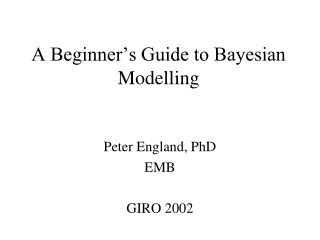DownloadDownload PresentationA Beginner’s Guide to Bayesian Modelling

# A Beginner’s Guide to Bayesian Modelling

Télécharger la présentation## A Beginner’s Guide to Bayesian Modelling

- - - - - - - - - - - - - - - - - - - - - - - - - - - E N D - - - - - - - - - - - - - - - - - - - - - - - - - - -
##### Presentation Transcript

1. A Beginner’s Guide to Bayesian Modelling Peter England, PhD EMB GIRO 2002

2. Outline • An easy one parameter problem • A harder one parameter problem • Problems with multiple parameters • Modelling in WinBUGS • Stochastic Claims Reserving • Parameter uncertainty in DFA

3. Bayesian Modelling: General Strategy • Specify distribution for the data • Specify prior distributions for the parameters • Write down the joint distribution • Collect terms in the parameters of interest • Recognise the (conditional) posterior distribution? • Yes: Estimate the parameters, or sample directly • No: Sample using an appropriate scheme • Forecasting: Recognise the predictive distribution? • Yes: Estimate the parameters • No: Simulate an observation from the data distribution, conditional on the simulated parameters

4. A One Parameter Problem • Data Sample [3,8,5,9,5,8,4,8,7,3] • Distributed as a Poisson random variable? • Use a Gamma prior for the mean of the Poisson • Predicting a new observation? • Negative Binomial predictive distribution

5. Poisson Example 1 – Estimation

6. Poisson Example 1 – Prediction

7. One Parameter Problem: Simple Case • We can recognise the posterior distribution of the parameter • We can recognise the predictive distribution • No simulation required • (We can use simulation if we want to)

8. Variability of a forecast • Includes estimation variance and process variance • Analytic solution: estimate the two components • Bayesian solution: simulate the parameters, then simulate the forecast conditional on the parameters

9. Main Features of Bayesian Analysis • Focus is on distributions (of parameters or forecasts), not just point estimates • The mode of posterior or predictive distributions is analogous to “maximum likelihood” in classical statistics

10. One Parameter Problem:Harder Case • Use a log link between the mean and the parameter, that is: • Use a normal distribution for the prior • What is the posterior distribution? • How do we simulate from it?

11. Poisson Example 2 – Estimation

12. Poisson Example 2 • Step 1: Use adaptive rejection sampling (ARS) from log density to sample the parameter • Step 2: For prediction, sample from a Poisson distribution with mean , with theta simulated at step 1

13. A Multi-Parameter Problem • From Scollnik (NAAJ, 2001) • 3 Group workers compensation policies • Exposure measured using payroll as a proxy • Number of claims available for each of last 4 years • Problem is to describe claim frequencies in the forecast year

14. Scollnik Example 1

15. Scollnik Example 1Posterior Distributions

16. Scollnik Example 1 • Use Gibbs Sampling • Iterate through each parameter in turn • Sample from the conditional posterior distribution, treating the other parameters as fixed • Sampling is easy for • Use ARS for

17. WinBUGS • WinBUGS is an expert system for Bayesian analysis • You specify • The distribution of the data • The prior distributions of the parameters • WinBUGS works out the conditional posterior distributions • WinBUGS decides how to sample the parameters • WinBUGS uses Gibbs sampling for multiple parameter problems

18. Stochastic Claims Reserving • Changes the focus from a “best estimate” of reserves to a predictive distribution of outstanding liabilities • Most stochastic methods to date have only considered 2nd moment properties (variance) in addition to a “best estimate” • Bayesian methods can be used to investigate a full predictive distribution, and incorporate judgement (through the choice of priors). • For more information, see England and Verrall (BAJ, 2002)

19. The Bornhuetter-Ferguson Method • Useful when the data are unstable • First get an initial estimate of ultimate • Estimate chain-ladder development factors • Apply these to the initial estimate of ultimate to get an estimate of outstanding claims

20. Conceptual Framework

21. Estimates of outstanding claims To estimate ultimate claims using the chain ladder technique, you would multiply the latest cumulative claims in each row by f, a product of development factors. Hence, an estimate of what the latest cumulative claims should be is obtained by dividing the estimate of ultimate by f. Subtracting this from the estimate of ultimate gives an estimate of outstanding claims:

22. The Bornhuetter-Ferguson Method Let the initial estimate of ultimate claims for accident year i be The estimate of outstanding claims for accident year i is

23. Comparison with Chain-ladder replaces the latest cumulative claims for accident year i, to which the usual chain-ladder parameters are applied to obtain the estimate of outstanding claims. For the chain-ladder technique, the estimate of outstanding claims is